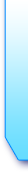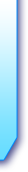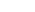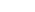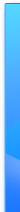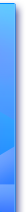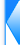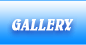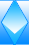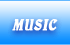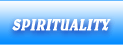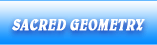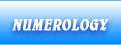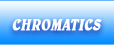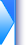### Search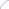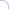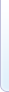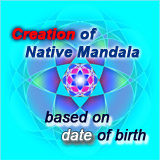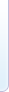exact phraseall wordsany word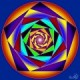Category >>  Sacred Geometry Pentagon - symbol of human perfection Pentagon pointing up is associated with the human body shape symbolizing human perfection. The top angle is the head and the relationship with the Divinity, the transcendence. The following lateral angles represent the hands, the control over the physical level and the action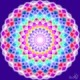Category >>  Numerology Golden number - universal Divine proportion get on the formula 1/a=a/1-a, otherwise writing 1/a-1=a, from this formula we want to find the ratio x=1/a, meaning x-1=1/x brings us to the equation x*x-x-1=0. The solution is 1,618… number that represents the golden number which can be found in the golden triangle of Pythagoras, the pentagon, the golden ellipse or spiral gold in the Fibonacci sequence which represents the natural growth. This special ratio is found almost everywhere in nature, in the human body and art. This is the principle that underlies the generation of fractals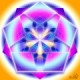Category >>  Numerology Five - the number of human perfection Five is the number of human perfection, the human body enroll in pointing up pentagon with five peaks. There are five senses (hearing, touch, sight, taste and smell), five major organs (brain, heart, liver, lungs and kidneys), five fingers on each hand and each foot. Five gives sharp intellect